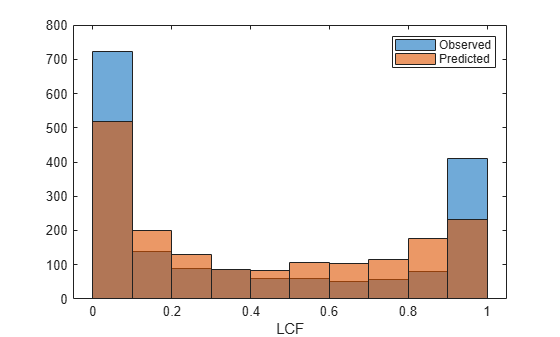# Regression

Create `Regression` model object for exposure at default

Since R2021b

## Description

Create and analyze a `Regression` model object to calculate the exposure at default (EAD) using this workflow:

1. Use `fitEADModel` to create a `Regression` model object.

2. Use `predict` to predict the EAD.

3. Use `modelDiscrimination` to return AUROC and ROC data. You can plot the results using `modelDiscriminationPlot`.

4. Use `modelCalibration` to return the R-square, RMSE, correlation, and sample mean error of the predicted and observed EAD data. You can plot the results using `modelCalibrationPlot`.

## Creation

### Syntax

``RegressionEADModel = fitEADModel(data,ModelType)``
``RegressionEADModel = fitEADModel(___,Name=Value)``

### Description

example

````RegressionEADModel = fitEADModel(data,ModelType)` creates a `Regression` EAD model object.```

example

````RegressionEADModel = fitEADModel(___,Name=Value)` specifies options using one or more name-value pair arguments in addition to the input arguments in the previous syntax. The optional name-value pair arguments set model object properties. For example, ```eadModel = fitEADModel(EADData,ModelType,PredictorVars={'UtilizationRate','Age','Marriage'},ConversionMeasure="ccf",DrawnVar='Drawn',LimitVar='Limit',ResponseVar='EAD')``` creates an `eadModel` object using a `Regression` model type. ```

### Input Arguments

expand all

Data for loss given default, specified as a table.

Data Types: `table`

Model type, specified as a string with the value of `"Regression"` or a character vector with the value of `'Regression'`.

Data Types: `char` | `string`

Name-Value Arguments

Specify optional pairs of arguments as `Name1=Value1,...,NameN=ValueN`, where `Name` is the argument name and `Value` is the corresponding value. Name-value arguments must appear after other arguments, but the order of the pairs does not matter.

Example: ```eadModel = fitEADModel(EADData,ModelType,PredictorVars={'UtilizationRate','Age','Marriage'},ConversionMeasure="ccf",DrawnVar='Drawn',LimitVar='Limit',ResponseVar='EAD')```

User-defined model ID, specified as `ModelID` and a string or character vector. The software uses the `ModelID` text to format outputs and is expected to be short.

Data Types: `string` | `char`

User-defined description for model, specified as `Description` and a string or character vector.

Data Types: `string` | `char`

Predictor variables, specified as `PredictorVars` and a string array or cell array of character vectors. `PredictorVars` indicates which columns in the `data` input contain the predictor information. By default, `PredictorVars` is set to all the columns in the `data` input except for the `ResponseVar`.

Data Types: `string` | `cell`

Response variable, specified as `ResponseVar` and a string or character vector. The response variable contains the EAD data and must be a numeric variable. By default, the `ResponseVar` is set to the last column of `data`.

Data Types: `string` | `char`

Boundary tolerance, specified as `BoundaryTolerance` and a positive scalar numeric. The `BoundaryTolerance` value perturbs the EAD response values away from 0 and 1, before applying a response transformation.

Data Types: `double`

Limit variable, specified as `LimitVar` and a string or character vector. `LimitVar` indicates which column in `data` contains the limit amount. The limit amount value in the `data` must be a positive numeric value. The limit depends on the loan. If its a credit card, the limit is the credit limit, and if this is a mortgage limit it is the initial loan amount. In general, `LimitVar` is the maximum amount that can be borrowed.

Note

`LimitVar` is required when `ConversionMeasure` is `'ccf'` or `'lcf'`. For more information on CCF and LCF, see Exposure at Default Regression Models.

Data Types: `string` | `char`

Drawn variable, specified as `DrawnVar` and a string or character vector. `DrawnVar` is the balance on the account at the time of observation, prior to default and EAD is the balance at the time of default. `DrawnVar` indicates which column in the `data` contains the drawn amount. The drawn variable value in the `data` can be a positive or negative numeric value.

Note

`DrawnVar` is required when `ConversionMeasure` is `'ccf'`.

If the `ConversionMeasure` is `'lcf'`, `DrawnVar` is not required. In this case, `DrawnVar` is set to `""`.

Data Types: `string` | `char`

Response transform, specified as `ConversionMeasure` and a character vector or string.

• `"ccf"` — Credit conversion factor (CCF) is the portion of the undrawn amount that will be converted into credit. The undrawn amount is the limit minus the drawn amount. The EAD thus becomes the drawn amount plus the CCF times the limit minus the drawn amount (```EAD = Drawn + CCF*(Limit - Drawn)```) .

• `"lcf"` — Limit conversion factor (LCF) is a fraction of the limit representing the total exposure. The EAD is then defined as the LCF times the limit (`EAD = LCF*Limit`).

For more information on CCF and LCF, see Exposure at Default Regression Models.

Data Types: `string` | `char`

## Properties

expand all

User-defined model ID, returned as a string.

Data Types: `string`

User-defined description, returned as a string.

Data Types: `string`

Underlying statistical model, returned as a compact linear model object. The compact version of the underlying regression model is an instance of the `classreg.regr.CompactLinearModel` class. For more information, see `fitlm` and `CompactLinearModel`.

Data Types: `CompactLinearModel`

Predictor variables, returned as a string array.

Data Types: `string`

Response variable, returned as a scalar string.

Data Types: `string`

Limit variable, returned as a string.

Data Types: `string`

Drawn variable, returned as a string.

Data Types: `string`

Boundary tolerance, returned as a scalar positive numeric.

Data Types: `double`

Conversion measure, returned as a string.

Data Types: `string`

Conversion transform, returned as a string that is `"complog"` if `ConversionMeasure` is `"ccf"` and `"logit"` when `ConversionMeasure` is `"lcf"`.

Data Types: `string`

## Object Functions

 `predict` Predict exposure at default `modelDiscrimination` Compute AUROC and ROC data `modelDiscriminationPlot` Plot ROC curve `modelCalibration` Compute R-square, RMSE, correlation, and sample mean error of predicted and observed EADs `modelCalibrationPlot` Scatter plot of predicted and observed EADs

## Examples

collapse all

This example shows how to use `fitEADModel` to create a `Regression` model for exposure at default (EAD).

```load EADData.mat head(EADData)```
``` UtilizationRate Age Marriage Limit Drawn EAD _______________ ___ ___________ __________ __________ __________ 0.24359 25 not married 44776 10907 44740 0.96946 44 not married 2.1405e+05 2.0751e+05 40678 0 40 married 1.6581e+05 0 1.6567e+05 0.53242 38 not married 1.7375e+05 92506 1593.5 0.2583 30 not married 26258 6782.5 54.175 0.17039 54 married 1.7357e+05 29575 576.69 0.18586 27 not married 19590 3641 998.49 0.85372 42 not married 2.0712e+05 1.7682e+05 1.6454e+05 ```
```rng('default'); NumObs = height(EADData); c = cvpartition(NumObs,'HoldOut',0.4); TrainingInd = training(c); TestInd = test(c);```

Select Model Type

Select a model type for `Regression` or `Tobit`.

`ModelType =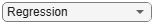"Regression";`

Select Conversion Measure

Select a conversion measure for the EAD response values.

`ConversionMeasure =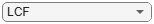"LCF";`

Create `Regression` EAD Model

Use `fitEADModel` to create a `Regression` model using `EADData`.

```eadModel = fitEADModel(EADData,ModelType,PredictorVars={'UtilizationRate','Age','Marriage'}, ... ConversionMeasure=ConversionMeasure,DrawnVar="Drawn",LimitVar="Limit",ResponseVar="EAD"); disp(eadModel);```
``` Regression with properties: ConversionTransform: "logit" BoundaryTolerance: 1.0000e-07 ModelID: "Regression" Description: "" UnderlyingModel: [1x1 classreg.regr.CompactLinearModel] PredictorVars: ["UtilizationRate" "Age" "Marriage"] ResponseVar: "EAD" LimitVar: "Limit" DrawnVar: "Drawn" ConversionMeasure: "lcf" ```

Display the underlying model. The underlying model's response variable is the transformation of the EAD response data. Use the `'BoundaryTolerance'`, `'LimitVar'`, and `'DrawnVar'` name-value arguments to modify the transformation.

`eadModel.UnderlyingModel`
```ans = Compact linear regression model: EAD_lcf_logit ~ 1 + UtilizationRate + Age + Marriage Estimated Coefficients: Estimate SE tStat pValue _________ _________ _______ __________ (Intercept) -2.4745 0.29892 -8.2781 1.6448e-16 UtilizationRate 6.0045 0.19901 30.172 7.703e-182 Age -0.020095 0.0073019 -2.752 0.0059471 Marriage_not married -0.03509 0.13935 -0.2518 0.8012 Number of observations: 4378, Error degrees of freedom: 4374 Root Mean Squared Error: 4.48 R-squared: 0.173, Adjusted R-Squared: 0.173 F-statistic vs. constant model: 305, p-value = 5.7e-180 ```

EAD prediction operates on the underlying compact statistical model and then transforms the predicted values back to the EAD scale. You can specify the `predict` function with different options for the `'ModelLevel'` name-vale argument.

```predictedEAD = predict(eadModel, EADData(TestInd,:),ModelLevel="ead"); predictedConversion = predict(eadModel, EADData(TestInd,:),ModelLevel="ConversionMeasure");```

For model validation, use `modelDiscrimination`, `modelDiscriminationPlot`, `modelCalibration`, and `modelCalibrationPlot`.

Use `modelDiscrimination` and then `modelDiscriminationPlot` to plot the ROC curve.

```ModelLevel =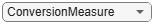"ConversionMeasure"; [DiscMeasure1, DiscData1] = modelDiscrimination(eadModel, EADData(TestInd,:),ModelLevel=ModelLevel); modelDiscriminationPlot(eadModel, EADData(TestInd, :), ModelLevel=ModelLevel,SegmentBy="Marriage");```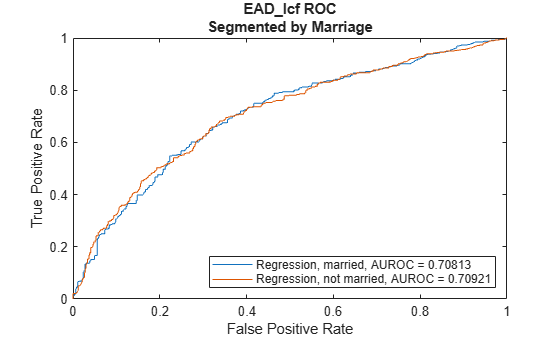Use `modelCalibration` and then `modelCalibrationPlot` to show a scatter plot of the predictions.

```YData =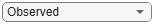"Observed"; [CalMeasure1, CalData1] = modelCalibration(eadModel, EADData(TestInd,:), ModelLevel=ModelLevel); modelCalibrationPlot(eadModel, EADData(TestInd,:),ModelLevel=ModelLevel,YData=YData);```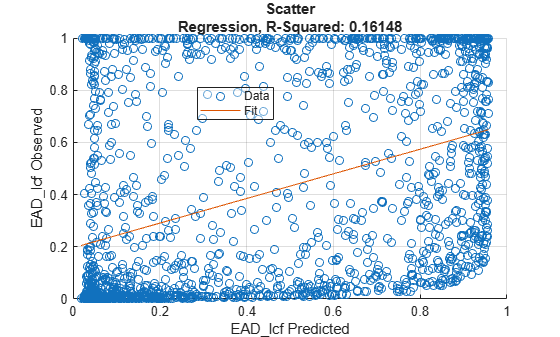Plot a histogram of observed with respect to the predicted EAD.

```figure; histogram(CalData1.Observed); hold on; histogram(CalData1.(('Predicted_' + ModelType))); xlabel(ConversionMeasure); legend('Observed', 'Predicted');```